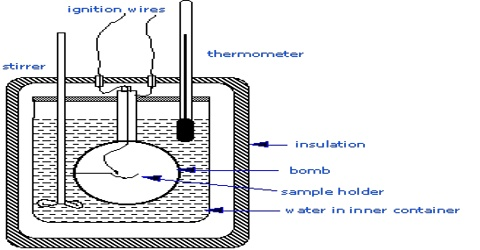# Heat of Reaction at Constant Pressure and at Constant Volume

At constant volume, the heat of reaction is equal to the change in the internal energy of the system. At constant pressure, the heat of reaction is equal to the enthalpy change of the system.

Heat of Reaction at Constant Pressure and at Constant Volume

When a reaction is carried out at constant volume as in a bomb calorimeter, the pressure within the calorimeter may change but no work is done. The heats of reaction in such cases correspond to ∆U. Ordinarily, reactions are carried out in open vessels under constant pressure conditions. ∆H and ∆U are, however, related by the equation

∆H = ∆U + P (V2 – V1)

= ∆U + P ∆V … … … (1)

It is clear from the above relation that ∆H and ∆U are equal if ∆V is zero, i.e., if there is no change in volume as a result of the reaction, but may differ if there is volume change. For reactions in which the reactants and products are either liquid or solid no appreciable change in volume takes place as a result of the reaction so that ∆H and ∆U are practically the same. This may not be so in the case of reactions involving gases.

The change in volume in a reaction due to the change in the number of moles of gas during the reaction as can be seen from the following:

For an ideal gas PV = nRT. If there are n1 moles of gaseous reactants and n2 moles of gaseous products we have,

P ∆V = PV2 – PV1 = n2RT – n2RT = (n2 – n1)RT = ∆n (RT)

where ∆n = n2 – n1; equation (1) may be written as

∆H = ∆U + ∆n (RT)

If n2 > n1; ∆H > ∆U; while if, n2 < n1; ∆n is negative and ∆H < ∆U

If in a reaction ∆V is positive, work is to be done by the system if it is a constant pressure process. More heat is consequently required making ∆H different from ∆U. When ∆V is negative in a constant pressure process, work is done on the system as the system contracts and heat is given out, again making ∆H and ∆U different.

The heat of Reaction at Constant Pressure:

The difference between the sum of enthalpies of products and the sum of enthalpies of reactants at a given temperature and constant pressure is called the heat of reaction at the constant pressure at a given temperature.

It is denoted by ΔH. Normally heat of reaction at constant pressure is specified at 298 K and 1 atm. Pressure. This heat of reaction is called the standard heat of reaction.

Thus at constant pressure heat of reaction is given by:

ΔH = ∑ΔHProducts – ∑ΔHReactants

ΔH is negative for exothermic reaction and positive for an endothermic reaction.

The heat of Reaction at Constant Volume:

The difference between the sum of internal energies of products and the sum of internal energies of reactants at a given temperature and constant volume is called the heat of reaction at the constant volume at a given temperature. It is denoted by ΔE.

ΔU = ΔH = ∑ΔUProducts – ∑ΔUReactants